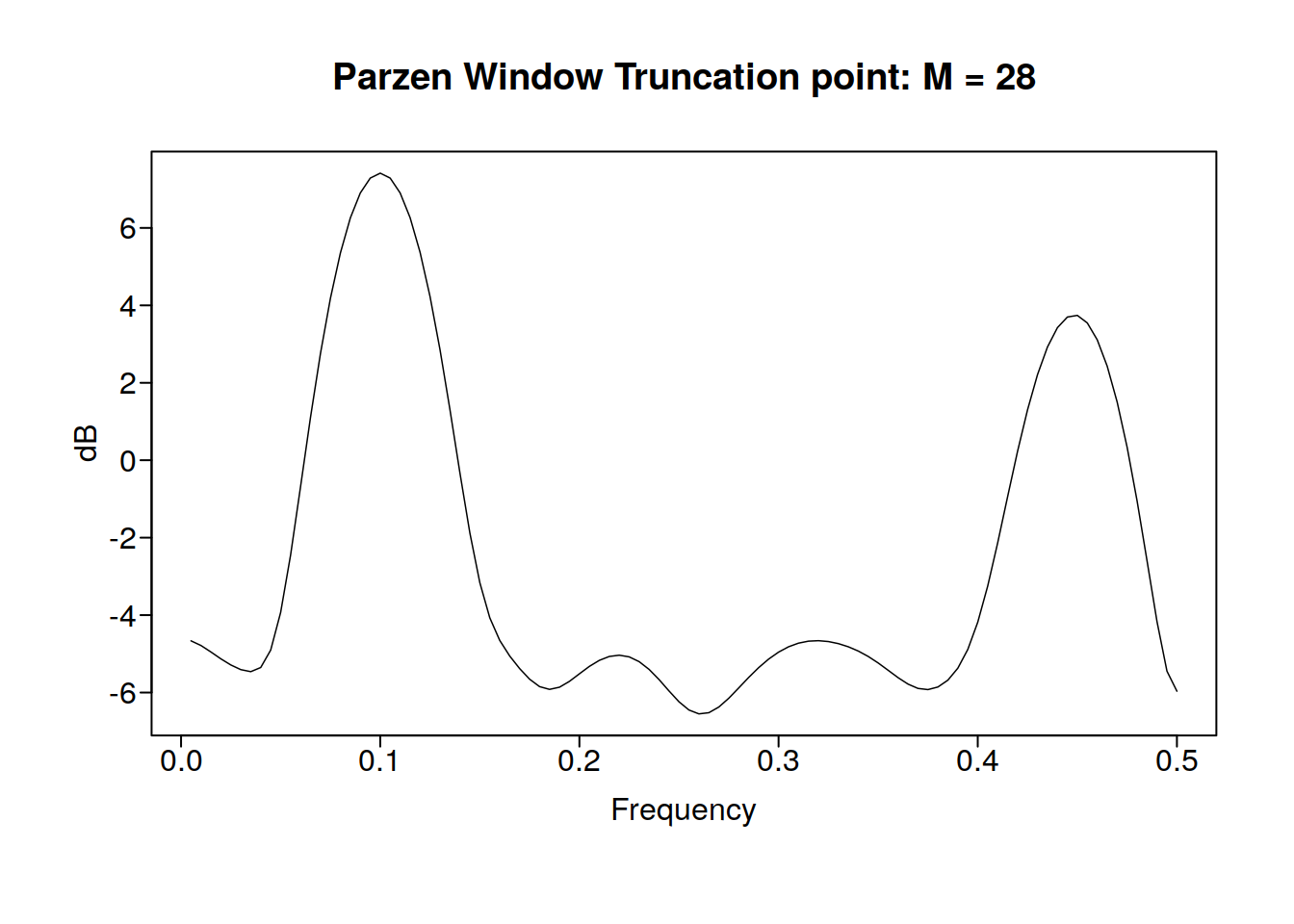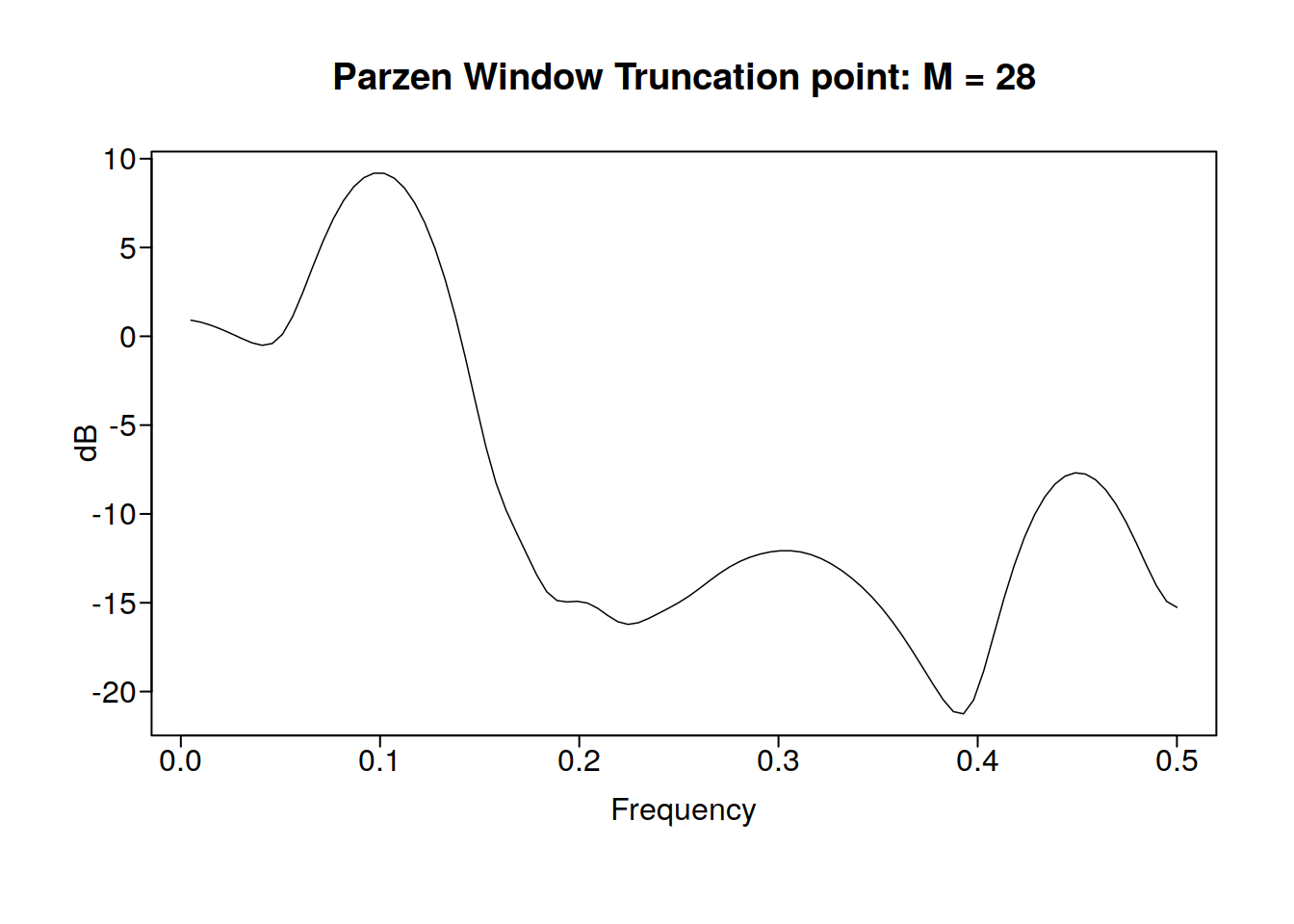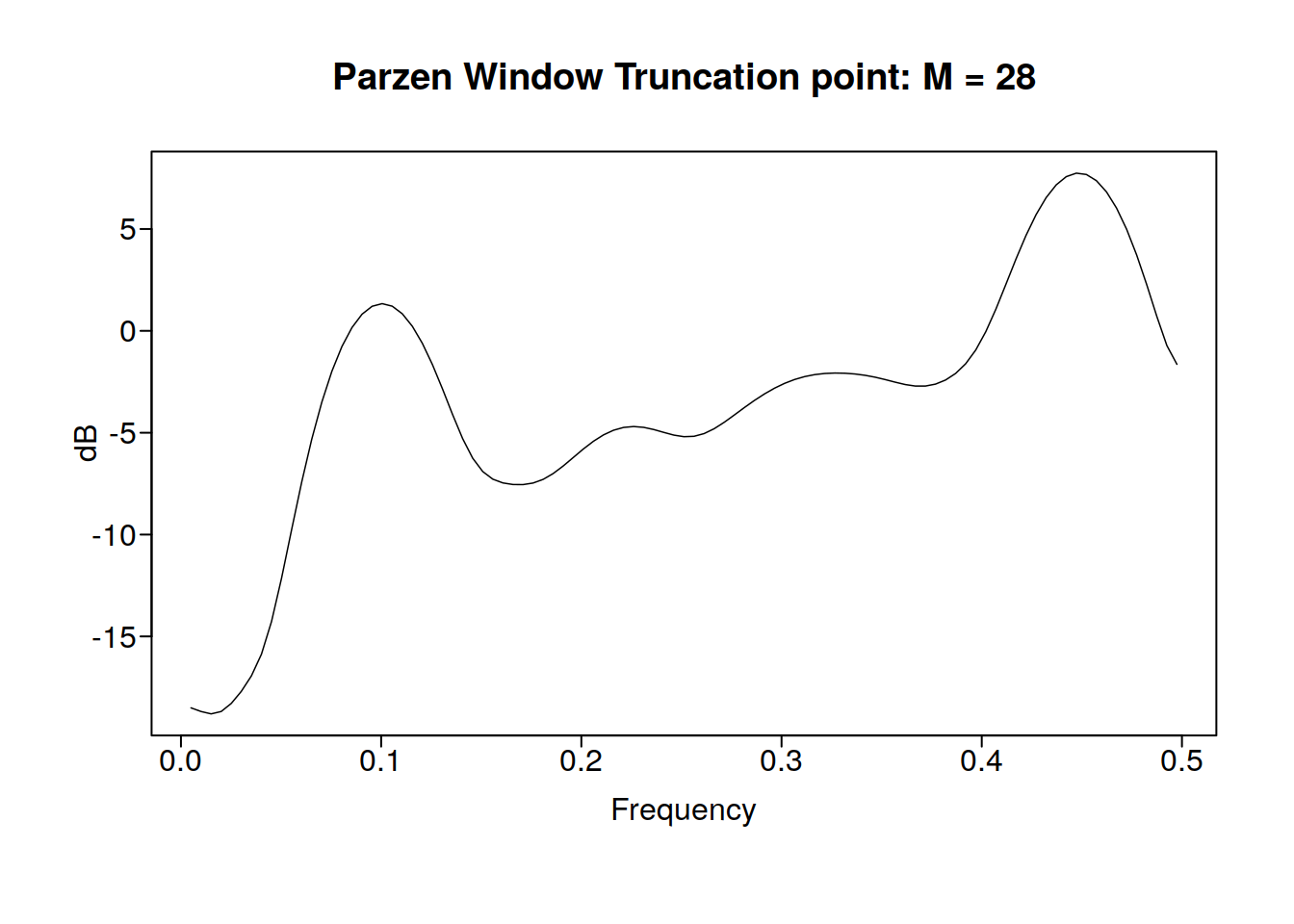# Unit 9 AR(1) Models and Filtering

## 9.1 Algebra review

Be able to find the roots of a 2nd order polynomial

$x = \frac{ - b \pm \sqrt {b^2 - 4ac} }{2a}$

$z = a + bi$ $i = \sqrt{-1}$ a is real b is imaginary $z^{*} = a - bi$ z* is complex conjugate

It is basically a vector so absolute value is just the magnitude

Unit circle is the values in the complex plane in which the magnitude of z is equal to one. If Z is a complex number with magnitude greater than one, it is outside the unit circle.

quad_form <- function(a, b, c) {
rad <- b^2 - 4 * a * c
if (is.complex(rad) || all(rad >= 0)) {
rad <- sqrt(rad)
} else {
rad <- sqrt(as.complex(rad))
}
round(cbind(-b - rad, -b + rad)/(2 * a), 3)
}

quad_form(c(1, 4), c(1, -5), c(6, 2))
##               [,1]          [,2]
## [1,] -0.500-2.398i -0.500+2.398i
## [2,]  0.625-0.331i  0.625+0.331i

## 9.2 Linear filters

A filter will turn $$Z_t$$ into $$X_t$$

for example:

$\mathrm{difference:} X_t = Z_t - Z_{t-1}$

Moving average also a filter (smoother)

### 9.2.1 Example

Consider $Z_1 = 8 , Z_2 =14, Z_3 = 14, Z_4 = 7$

If we apply the difference filter to this, we have that: $X_2 = 6, X_3 = 0, X_4 = 7$

Note: the differenced data are a realization of length n-1

We can use the difference filter to remove the wandering behjaviior

### 9.2.2 5 point moving average

We can only get a realization of n-4. (n-nopoints-1). What does the 5 point moving average do (it averages a point and two points ahead and two behind). This filter can filter out some frequencies

## 9.3 Types of filters:

• Low pass filter
• Filters out high frequency
• Such as 5 point moving average, it smooths
• High pass filter
• Leaves high freq but removes low freq
• Such as differencing

### 9.3.1 An example in R

mafun <- function(xs, n) {
stats::filter(xs, rep(1, n))/n
}
dfun <- function(xs, n) {
diff(xs, lag = n)
}
th <- ggthemes::theme_few()
data(fig1.21a)
ma <- mafun(fig1.21a, 5)
d <- dfun(fig1.21a, 1)
fp <- tplot(fig1.21a) + th
mp <- tplot(ma) + th
dp <- tplot(d) + th

And here lets view the 5 point moving average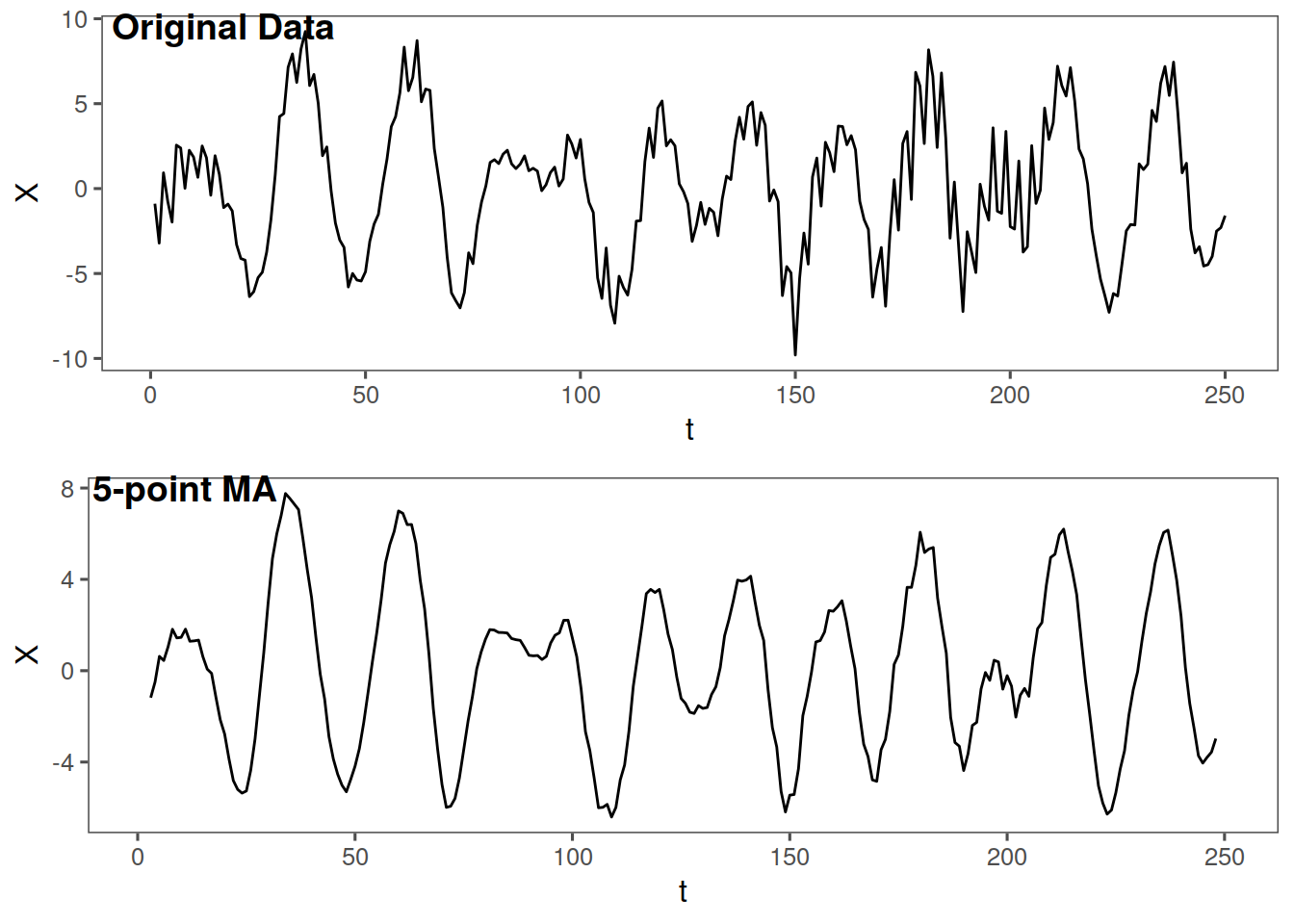And now a look at the difference filter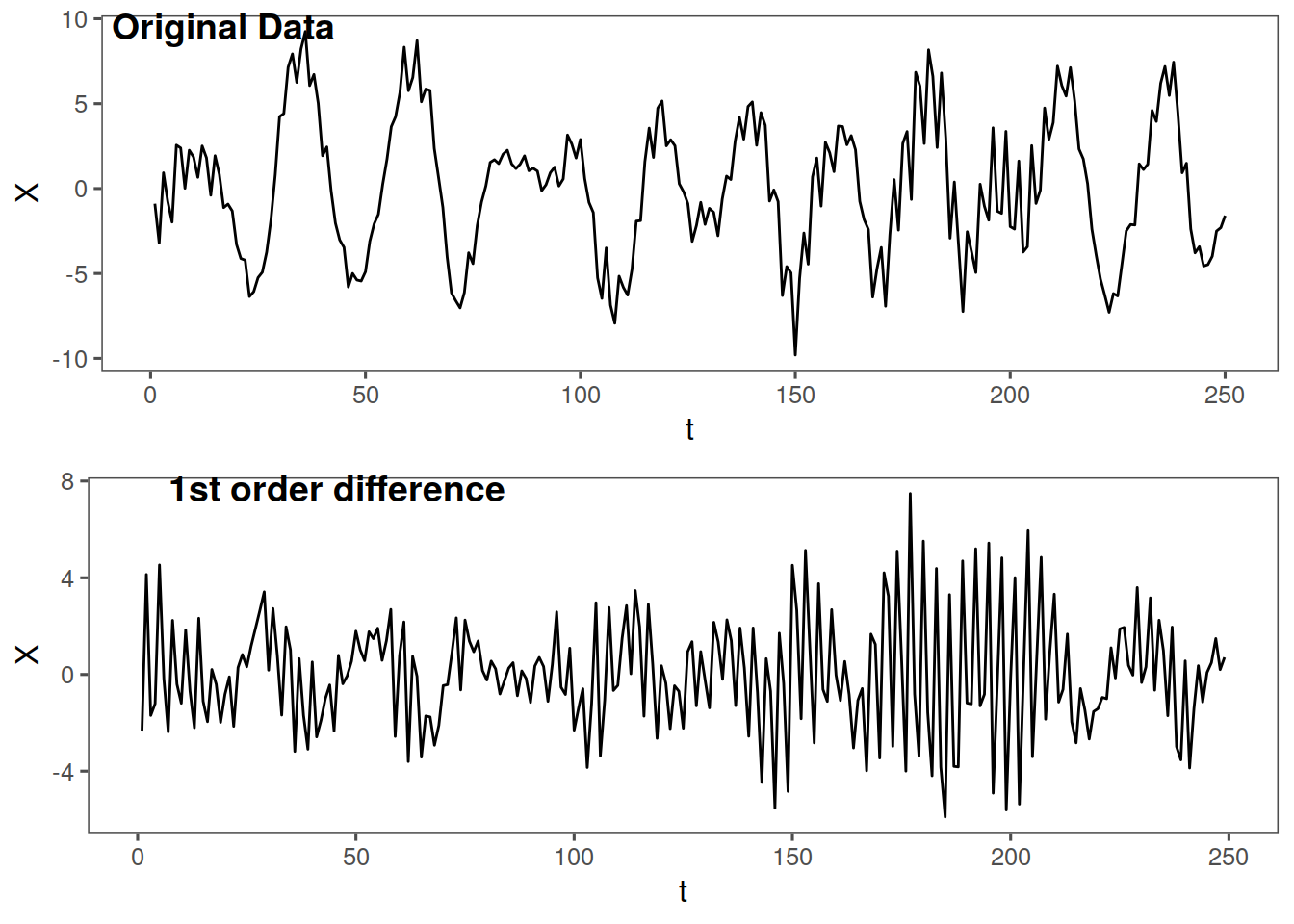### 9.3.2 An another example

rlz <- gen.sigplusnoise.wge(200, coef = c(5, 3), freq = c(0.1, 0.45), vara = 10,
sn = 1, plot = F)

#### 9.3.2.1 Some plots

pfun <- function(x, n, l) {
fp <- tplot(x) + th
mp <- tplot(mafun(x, n)) + th
dp <- tplot(dfun(x, l)) + th
list(original = fp, MA = mp, dif = dp)
}
plts <- pfun(rlz, 5, 1)
plts$original plts$MA
plts\$dif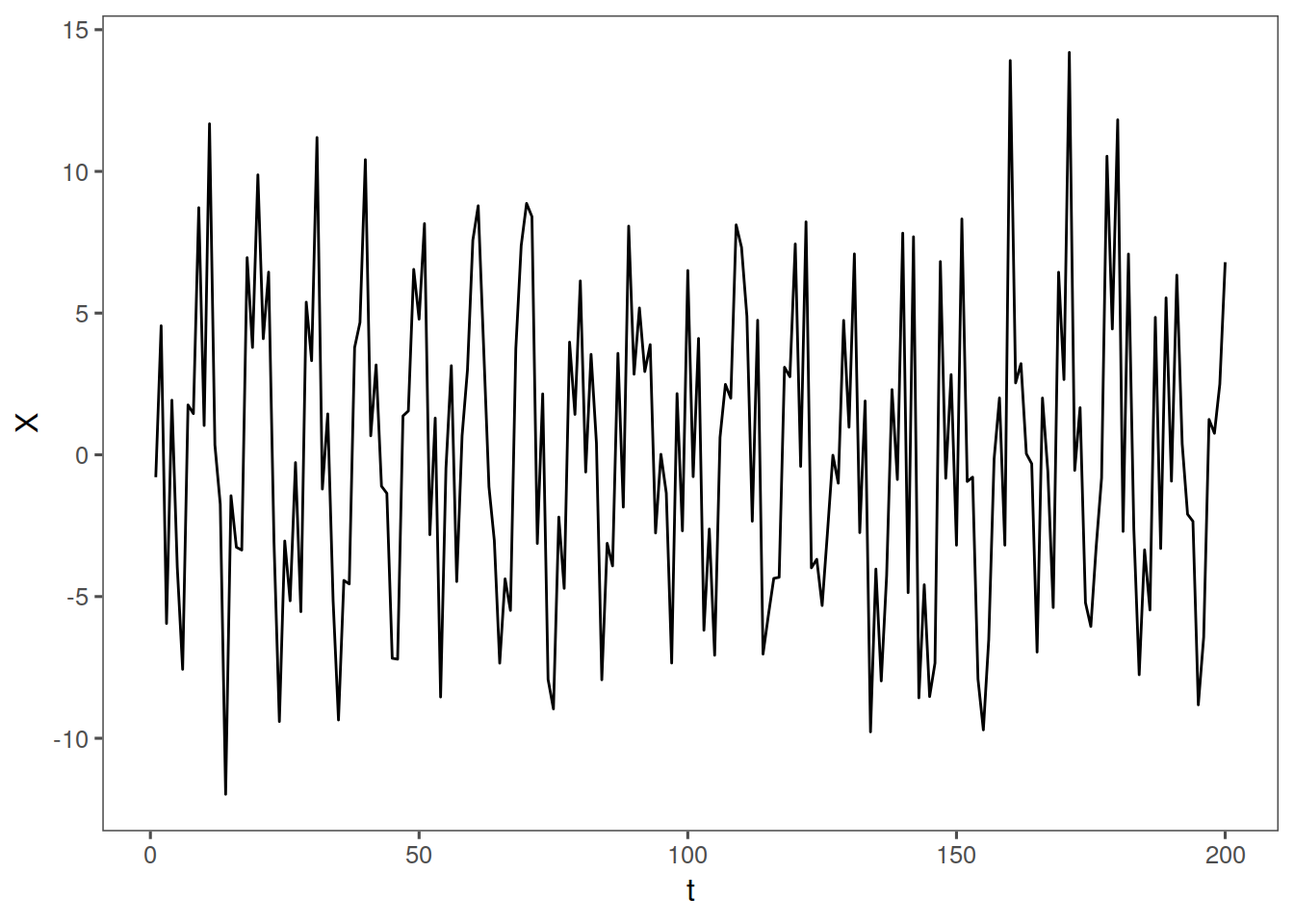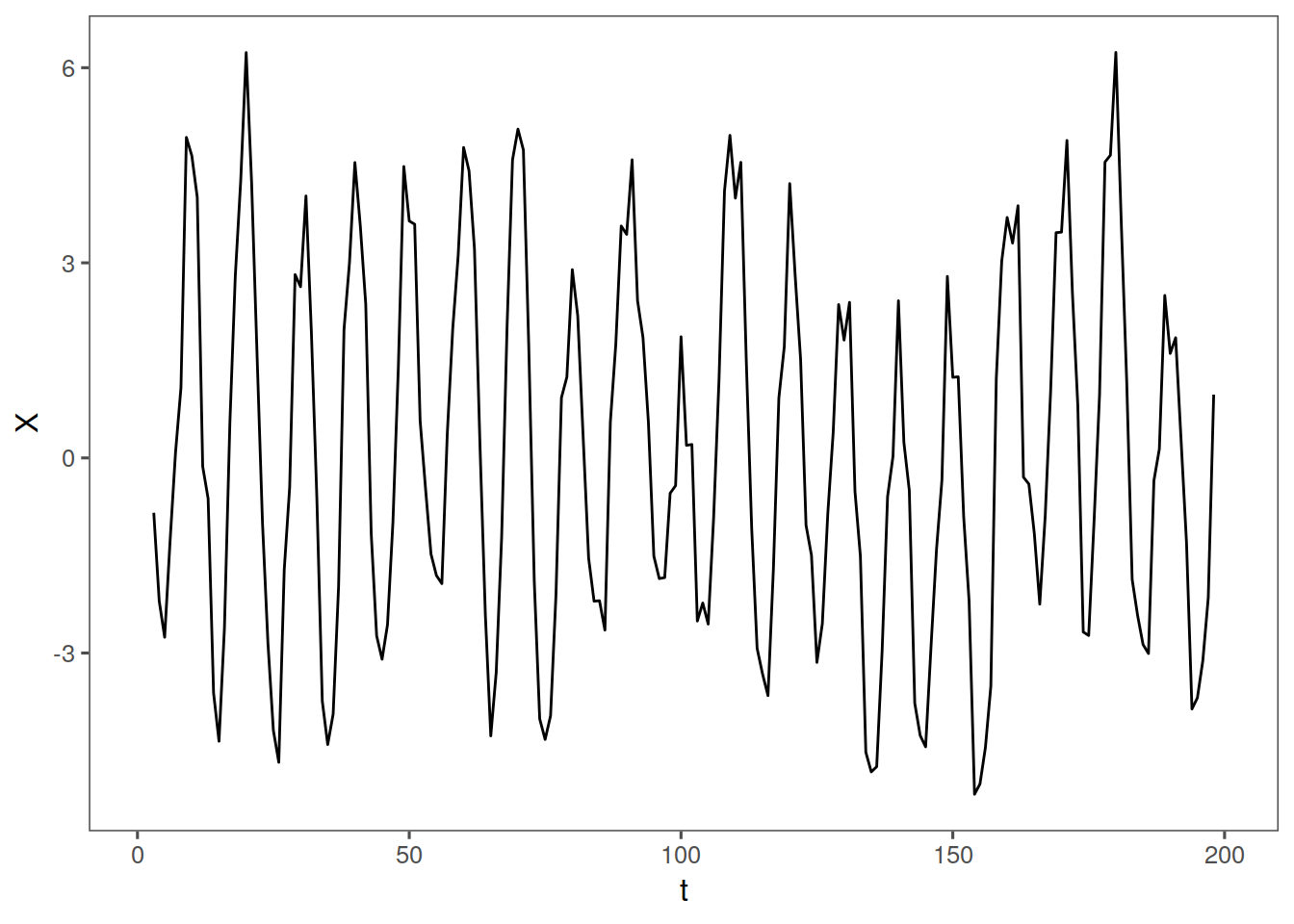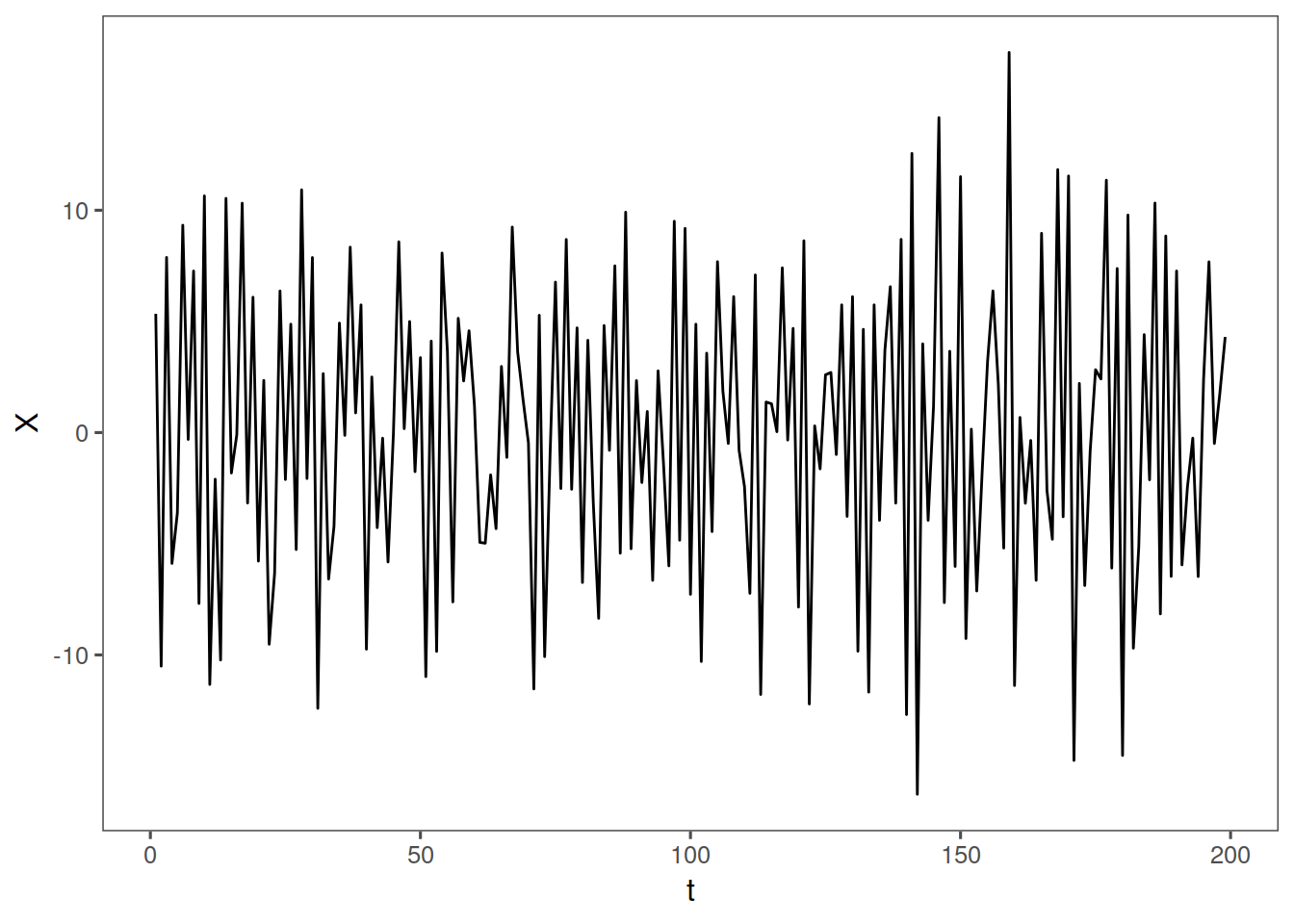#### 9.3.2.2 Some Parzen Windows

parzen.wge(rlz)
parzen.wge(na.omit(mafun(rlz, 5)))
parzen.wge(na.omit(dfun(rlz, 1)))